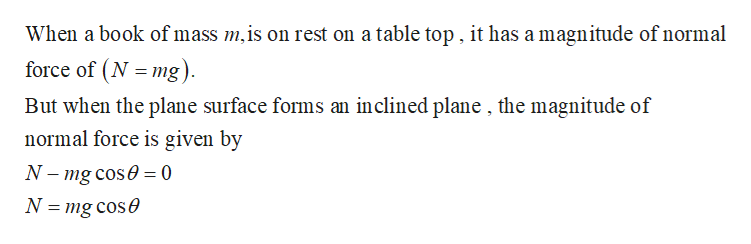# Consider the normal force on a book at rest on a tabletop. If the table is tilted so that the surface forms an inclined plane, will the magnitude of the normal force change? If so , how?

Question
29 views

Consider the normal force on a book at rest on a tabletop. If the table is tilted so that the surface forms an inclined plane, will the magnitude of the normal force change? If so , how?

check_circle

Step 1

The below figure represents the free body diagram of the book on an inclined plane .

Step 2help_outlineImage TranscriptioncloseWhen a book of mass m,is on rest on a table top , it has a magnitude of normal force of (N = mg). But when the plane surface forms an inclined plane , the magnitude of normal force is given by N- mg cose = 0 N = mg cose fullscreen

### Want to see the full answer?

See Solution

#### Want to see this answer and more?

Solutions are written by subject experts who are available 24/7. Questions are typically answered within 1 hour.*

See Solution
*Response times may vary by subject and question.
Tagged in

### Physics